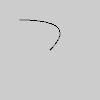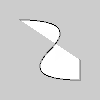Specifies a `Py5Shape` object’s vertex coordinates for quadratic Bezier curves.

## Examples#```def setup():
py5.size(100, 100, py5.P2D)
s = py5.create_shape()
s.begin_shape()
s.no_fill()
s.vertex(20, 20)
s.end_shape()
py5.shape(s)
``````def setup():
py5.size(100, 100, py5.P2D)
s = py5.create_shape()
s.begin_shape()
s.vertex(20, 20)
s.vertex(80, 60)
s.end_shape()
py5.shape(s)
```

## Description#

Specifies a `Py5Shape` object’s vertex coordinates for quadratic Bezier curves. Each call to `quadratic_vertex()` defines the position of one control point and one anchor point of a Bezier curve, adding a new segment to a line or shape. The first time `quadratic_vertex()` is used within a Py5Shape.begin_shape() call, it must be prefaced with a call to Py5Shape.vertex() to set the first anchor point. This method must be used between Py5Shape.begin_shape() and Py5Shape.end_shape() and only when there is no `MODE` parameter specified to Py5Shape.begin_shape().

Drawing 2D bezier curves requires using the `P2D` renderer and drawing 3D bezier curves requires using the `P3D` renderer. When drawing directly with `Py5Shape` objects, bezier curves do not work at all using the default renderer.

## Signatures#

```quadratic_vertex(
cx: float,  # the x-coordinate of the control point
cy: float,  # the y-coordinate of the control point
cz: float,  # the z-coordinate of the control point
x3: float,  # the x-coordinate of the anchor point
y3: float,  # the y-coordinate of the anchor point
z3: float,  # the z-coordinate of the anchor point
/,
) -> None